# ICSE Solutions for Chapter 9 Matrices Class 10 Mathematics

Question 1: Find x and y, if:Solution 1:Comparing the corresponding elements, we get,
6x - 10 = 8
⇒ 6x = 18
⇒ x = 3
-2x + 14 = 4y
⇒ 4y = -6 + 14 = 8
⇒ y = 2

Question 2: Find x and y if :
(i) 3[3  x] + 2[y  -3] = [10  0]
(ii)Solution 2:
(i) 3[4  x] + 2[y  -3] = [10  0]
[12  3x] + [2y  -6] = [10  0]
[12+2y  3x–6] = [10  0]
Comparing the corresponding elements, we get,
12 + 2y = 10 and 3x - 6 = 0
Simplifying, we get, y = -1 and x = 2.

(ii)Comparing the corresponding the elements, we get,
-x + 8 = 7 and 2x – 4y = -8
Simplifying, we get,
x = 1 and y = 5/2 = 2.5

Question 3: Solve for a, b and c if
(i)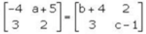(ii)Solution 3:
If two matrices are equal, then their corresponding elements are also equal.
(i) a + 5 = 2 ⇒ a = -3
-4 = b + 4 ⇒ b = -8
2 = c – 1 ⇒ c = 3

(ii) a = 3
a - b = -1
⇒ b = a + 1 = 4
b + c = 2
⇒ c = 2 – b = 2 – 4 = -2

Question 4:find A.

Solution 4:Question 5:(i) (AB)C
(ii) A(BC)
Is A(BC) = (AB)C ?

Solution 5:Hence, A(BC) = (AB)C.

Question 6: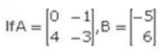and 3A x M = 2B; find matrix M.

Solution 6:
Let the order of matrix M be a×b.
3A × M = 2B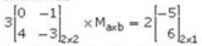Clearly, the order of matrix M is 2 x 1.Comparing the corresponding elements, we get,
- 3y = -10
⇒ y = 10/3
12x - 9y = 12
⇒ 12x - 30 = 12
⇒ 12x = 42
⇒ x = 7/2Question 7:Solution 7: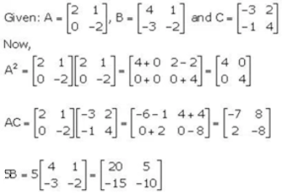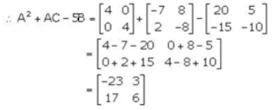Question 8: Given: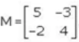find its transpose matrix Mt. If possible, find:
(i) M + M
(ii) M- M

Solution 8:Question 9:Solution 9: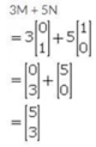Question 10: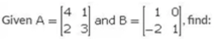(i) A - B
(ii) A2
(iii) AB
(iv) A - AB + 2B

Solution 10: